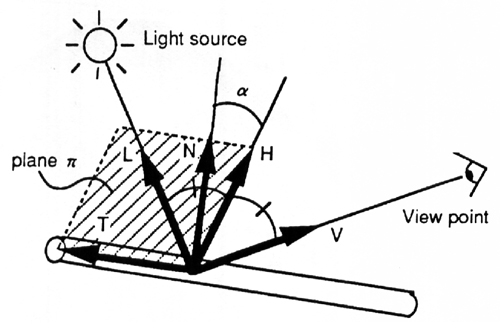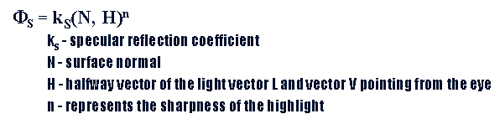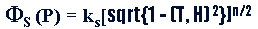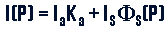Rendering TechniqueNow that we have created a hair model and have modeled the dynamic behavior of the hair, how do we render the hair. To render the hair we must assume that a hair segment is a cylinder with a very small radius. By doing so we are able to use Blinn's specular model.The specular component of the hair is represented by taking N on the Pi plane spanned by H and T. Where T is the hair cylinder's tangent. (N,H) is then easily calculated by taking the cosine of the angle beta. This in turn equals the square root of 1 - (T, H)^2. The specular term at P is defined as. The toal intensity at a point P is given as. Where I_s is the intensity of ambient light, k_s is the ambient reflection coefficient and I_s is the intensity of the light source. We are now able to render the hair in an efficient manner.